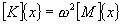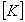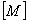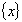# Strand7 natural frequency solver

The natural frequency solver is used to calculate the natural frequencies (or free vibration frequencies) and corresponding vibration modes of an undamped structure. The natural frequency analysis problem, is formulated as the following eigenvalue problem:whereGlobal stiffness matrixGlobal mass matrixVibration mode vectorNatural (circular) frequency (radians/sec)

The natural frequency solver performs the following steps:

• Calculates and assembles the element stiffness and mass matrices to form the global stiffness and mass matrices. In the stiffness calculation, material temperature dependency is considered through the user nominated temperature case. Either a consistent or lumped mass matrix can be used according to the solver option setting. The geometric stiffness matrix will be formed and assembled to the global stiffness matrix when an initial solution is applied. Constraints are assembled in this process.

If the initial file is from a nonlinear solution, the stiffness and mass matrices calculation will be based on the current material status and geometry. More specifically, the current material modulus values will be used for nonlinear elastic material. For plastic material, the initial modulus is used. The current geometry is used if geometric nonlinearity is considered in the initial solution.

• Modifies the stiffness matrix if a shift value is applied. A shift may be used to determine modes near to a desired value.

• Solves the eigenvalue problem to get frequencies and the corresponding mode shapes using the Sub-Space Iteration Method.

### Notes

1. To help the user visualise the results, stress and strain patterns may be generated. As the results are patterns only, only the relative magnitude is meaningful.

2. Frequency shift can be used to calculate higher modes by excluding lower modes. A special application of the shift is in the analysis of unconstrained structures. If the solver detects rigid body motion, a shift of -1.0 Hz is automatically applied and a message given.

3. The Sturm Sequence check is an effective method for checking the convergence of the sub-space iteration method to ensure that the eigenvalue solution has converged and no eigenvalues are missed.

For more information on natural frequency analysis, see Strand7 Webnotes - Linear / Dynamics.